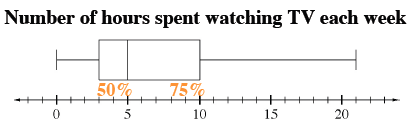### Home > MC2 > Chapter 5 > Lesson 5.2.2 > Problem5-60

5-60.
1. Elvin found the box-and-whisker plot below in the school newspaper. Homework Help ✎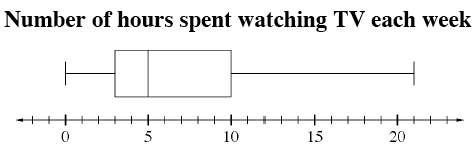1. Based on the plot, what percent of students watch more than 10 hours of television each week?

2. Based on the plot, what percent of students watch less than 5 hours of television each week?

3. Can Elvin use the box-and-whisker plot to find the mean (average) number of hours of television students watch each week? If so, what is it? Explain your reasoning.

4. Can he use the plot to find the median? If so, what is it? Explain your reasoning.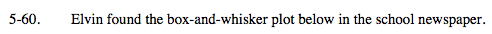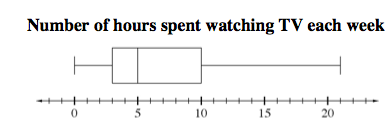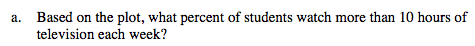The box plot illustrates the quartiles of the data represented by the box in the middle.

If the right side of the box over the 10th hour represents the 3rd quartile of the data or the 75th percentile, what percent of students watch more than 10 hours of television each week?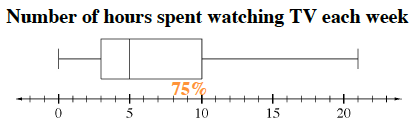25%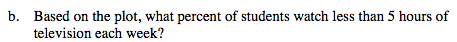If the middle line of the box represents the middle half of all the data, what percent
of students watch less than 5 hours of television each week?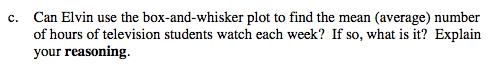Box plots are useful for representing the distribution of data, but they do not express any specific data other than the minimum and maximum.

No, because individual data points are not given.

Since the mean would be the average number of hours that students watched television each week, could we calculate the mean with the information given or are specific data points necessary? Remember, mean and median are not the same.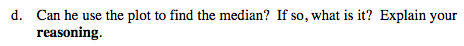The median is the middle half of all the data. Where on the box plot can this be represented? Does this make sense?